# RBSE Solutions For Class 10 Science Chapter 6: Chemical Reaction and Catalyst | Textbook Important Questions & Answers

RBSE Class 10 Science Chapter 6 Chemical Reaction and Catalyst Solutions is the most useful resource for the students to get a general overview of the topics taught in class from Chapter 6 of Class 10 Science textbook. It is seen that everyday substances change forms. These can be physical or chemical changes. Chapter 6 of RBSE Class 10 Science deals with concepts such as physical and chemical change, chemical equation, neutralization, catalyst and so on. Mastering these concepts and topics can be challenging and interesting. We have compiled here the best resource to help the students prepare most competently for the board exams. RBSE Class 10 Solutions of Chapter 6 Science, Chemical Reaction and Catalyst will help the students to learn the concepts and master the subject thoroughly.

We have given here the list of chapter-wise questions and solutions from the Class 10 Chapter 6 Science of RBSE. These questions will discuss all the main topics from the chapter and also consists of solutions to the questions that are asked at the end of the chapter in the textbook.

## Rajasthan Board Class 10 Science Chapter 6- BYJU’S Important Questions & Answers

### RBSE Class 10 Science Chapter 6 Objective Questions-Important Questions and Solutions

1. Conversion of FeCl2 to FeCl3 is called _________

(a) Oxidation

(b) Reduction

(c) Decomposition

(d) Combination

2. A substance breaks in two simple small molecules, then reaction is called ____

(a) Dissociation

(b) Displacement

(c) Oxidation

(d) Reduction

3. Substances which donate electron are called________

(a) Oxidizing agent

(b) Catalyst

(c) Reducing agent

(d) None of these

4. Reactions which proceed in both directions________

(a) Oxidation

(b) Reduction

(c) Irreversible

(d) Reversible

5. Those which increase rate of reaction_______

(a) Catalyst

(b) Oxidizing Agent

(c) Reducing agent

(d) None of these

6. Enzymes are__________________

(a) Negative catalyst

(b) Positive catalyst

(c) Autocatalyst

(d) Bio-catalyst

7. 2Mg2 + O → 2MgO, in this reaction Magnesium metal is____________

(a) Oxidized

(b) Reduced

(c) Dissociated

(d) Displaced

8. Which sign is used for reversible reaction?

9. The reaction which is catalysed by the product formed______

(a) Biochemical

(b) Reversible

(c) Autocatalysed

(d) Irreversible

10. In exothermic reaction, heat is _______

(a) released

(b) absorbed

(c) Soluble

(d) None of these

### RBSE Class 10 Science Chapter 6 Very Short Answer Questions-Important Questions and Solutions

1. What is chemical change?

Answer: Change in which the chemical properties and the composition of substance change and form new substances is called chemical change. During the process of chemical change, it is not necessary that removing the reason for change will help to obtain the original substance again. Example: On burning Coal, Carbon dioxide is obtained. However, Coal cannot be obtained from C02.

2. Name the catalyst which changes vegetable oil to vegetable ghee.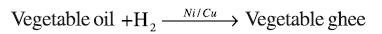, Ni or Nickel is the heterogeneous catalyst used, while cu is the catalyst promoter.

3. How many types of catalysts are there? Write their names?

Answer: On the basis of physical state there are two main types of catalysts, known as homogeneous and heterogeneous catalysts. Now, there are also 4 types of catalyst on the basis of action. They are positive catalyst, negative catalyst, auto-catalyst and bio-catalyst.

4. Zn + CuS04 → ZnS4 + Cu: This is an example of which type of reactions?

Answer: Zn + CuS04 → ZnS4 + Cu is the example of a displacement reaction. In a displacement reaction, a more reactive element displaces a comparatively less reactive element. Zn is a more reactive element and Cu is less reactive. Hence, Zn displaces Cu from CuS04.

5. Give an example of redox reaction.

Answer: 2Mg + O2 → 2MgO is the example of a redox reaction. In this reaction, Mg is oxidised and oxygen is reduced.

6. What are reversible reactions?

Answer: The reactions in which the reactants react to produce products under the same conditions,while the products also react to form reactants are called reversible reactions. In these, reactions happen in both the directions. Reversible reactions are divided into two reactions, the forward reaction and the backward reaction that occur simultaneously.

7. What is the work of catalyst promoter and catalyst inhibitor?

Answer: Catalyst promoters are substances that when added to the catalyst in the reaction mixture, boosts the activity of the catalyst. They only increase the activity of catalysts and are not catalysts themselves. Meanwhile, catalyst inhibitors are substances that, when mixed in the reaction mixture, decrease the activity of the catalyst.

8. What is the reaction between acid and base called?

Answer: When acid and base reacts, salt and water are formed. This reaction is called Neutralization.

9. How many types of reactions are there on the basis of velocity?

Answer: Chemical reactions are two on the basis of velocity. They are fast reactions and slow reactions.

10. Write an example of thermal dissociation reaction.

Answer: Thermal dissociation reaction is a chemical reaction, in which a substance decomposes reversibly, when the temperature is raised. When water is heated it decomposes into hydrogen and oxygen.

2H20⇌ 2H2+02..

11. What is the work of a catalyst in any reaction?

Answer: The catalyst alters the velocity of the chemical reaction, however it itself remains unchanged.

12. What is the fundamental principle of balancing a chemical equation?

Answer: Here, the chemical law of fundamental combination is applied as the principle of balancing a chemical equation. As per the law, in a chemical reaction, whatever is the mass of the reactant, the same product of same mass is created, which means that the mass is conserved in complete reaction. In a chemical reaction, mass is neither created nor destroyed. Hence, the number of atoms of the reactants and the products are the same on both sides of the arrow. Equations are balanced by increasing or reducing the number of molecules on both sides.

13. What is a redox reaction?

Answer: Reactions in which substances react with oxygen is called oxidation reaction, while reactions in which oxygen is removed is called reduction reaction. A chemical reaction in which both oxidation and reduction takes place simultaneously is called a redox reaction. In this, oxidation-reduction half reactions occur. One substance donates the electron, while the other substance accepts the electron. In this one substance is oxidised, while the other is reduced.

14. What type of reaction is burning of coal?

Answer: When coal is burnt to produce energy, the atoms in the carbon combine with oxygen from the air to make CO2, thus making the burning of coal is a non-reversible chemical change. Since, both oxidation and reduction takes place in this reaction, this is an example for redox reactions.

15. What will be the pH of a solution, when a strong acid and a strong base react?

Answer: When a strong acid and a strong base of the same concentration react then the pH of a solution is 7

### RBSE Class 10 Science Chapter 6 Short Answer Questions-Important Questions and Solutions

16. Write the difference between a physical and chemical reaction.

Answer: GIven here are some of the properties of a physical and chemical reaction. See, how they are different from each other:

 Physical Reaction Chemical Reaction Only physical properties like smell, colour, state etc., undergo change Substance formed as a result of the chemical reaction have completely different chemical properties and composition from the reactants Original substance can be obtained by removing the cause of change Initial substance cannot be obtained Temporary change Permanent change New substance is not formed New substance is formed

17. Write one example of a combination and decomposition reaction.

Answer: The chemical reaction in which two or more substances combine to form a single substance, is known as combination reaction. In this reaction, new bonds are produced between the reactants. As the reactants just add in this reaction, it is called combination and addition reaction.

Example of this reaction is burning coal: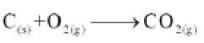Meanwhile, when a reactant disassociates to provide two or more products, the reaction is called a dissociation reaction.

Example: Using electrolysis to disassociate water

2H2O

18.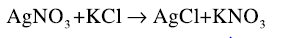What type of reaction is this? Write the name and explain.

Answer: This is an example for double replacement reaction. In this reaction, atoms or group of atoms of both reactants are displaced mutually to form a new component. For example, silver displaces potassium to form AgCl(Silver Chloride), while potassium displaces silver to form KNO3. (Potassium Nitrate).

19. Explain oxidation and reduction on the basis of electronic charge.

Answer: Reaction in which an atom, ion or molecule donates an electron (e), is called Oxidation. For example, in the equation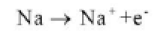, sodium donates electron (e) and oxidises to Na+ cation. In an oxidation reaction, a neutral atom changes to a cation or charge on cation (positive ion) increases or charge on negative ion decreases. Similarly, reactions in which atom, ion or molecule accepts an electron (e) is a reduction reaction. Example of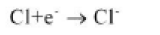where chlorine accepts an electron (e), and gets reduced to chloride ion (cl). In reduction reaction, neutral atoms accept an electron (e) and form an anion. Here, the charge on anion increases or charge on cation decreases.

20. What are the types of catalysts? Write their name.

Answer: On the basis of physical state, catalysts are of two main types. 1) Homogeneous Catalyst-If in a chemical reaction, the catalyst, reactants and products are in the same physical state, then it is called a homogeneous catalyst. 2) Heterogeneous catalyst-If in a chemical reaction, the physical state of the catalyst and the reactants are different, then the catalyst is a heterogeneous catalyst. Now, catalysts are also categorised on the basis of action. 1) Positive Catalyst-A catalyst that increases the rate of chemical reactions are called positive catalysts. 2) Meanwhile, catalysts that reduce the rate of chemical reaction are known as negative catalysts. 3) Auto-catalysts- When a product created in a chemical reaction itself acts as a catalyst, it is called an auto catalyst. 4) Finally, there is the bio-catalyst, which are the substances used to increase the velocity of biochemical reactions.

21. Explain the types of dissociation reactions.

Answer: A chemical reaction under similar conditions, where one single molecule is broken down into multiple simpler substances is called dissociation or decomposition reaction. Click to check more details about the decomposition or dissociation reaction.

22. Why is some amount of ethyl alcohol added in chloroform?

Answer: Chloroform has a tendency to react with the oxygen from hair, thus resulting in the manufacture of a poisonous gas, phosgene. Adding ethyl alcohol to chloroform helps to prevent this reaction. It also helps to reduce the speed of the reaction.

23. Aqueous solution of salt formed by weak acid and strong base is alkaline. Why?

Answer: When an acid and base reacts than salt and water is created. Strong acid and strong base becomes completely ionised. However, in a neutralization reaction between the weak acid and a strong base, the acid does not completely dissociate or ionise. Some amount of it remains in the undissociated form. If a solution has an equal amount of base and acid OH ions will be more in the solution and pH of the solution will be more than 7. The salt of the weak acid and strong base undergoes electrolysis to produce more OH- ions.

See the equation of sodium acetate getting hydrolyzed in water.

Na(+) + CH3COO(-) + H2O = Na(+) + CH3COOH + OH(-).

Seeing as acetic acid is a weak acid it remains unionised in water and OH- ions will make the solution basic or alkaline.

24. Are these reactions possible? Write an answer with reason.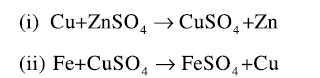Answer: In displacement reactions, more reactive elements displace comparatively less reactive elements.

(i) This reaction is not possible as copper is less reactive than zinc so it will not displace zinc.

(ii) This reaction is possible as iron is more reactive than copper. Iron will be able to replace copper.

25. Identify the oxidation-reduction in the following reactions.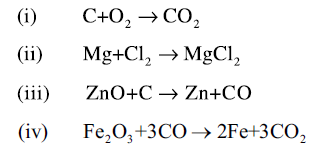Answer: (i) Oxidation of carbon and reduction of oxygen takes place in this chemical reaction

(ii) Oxidation of magnesium and reduction of chlorine occurs in this equation

(iii) In this reaction, reduction of Zinc oxide and oxidation of carbon happens

(iv) Reduction of iron oxide and oxidation of carbon monoxide happens

### RBSE Class 10 Science Chapter 6 Essay Questions-Important Questions and Solutions

26. How many types of chemical reactions are there?

Answers: When a chemical change takes place in a substance, the chemical composition and properties of the products differ from the original substances. This chemical change in any substance is called chemical reactions. In a chemical reaction, the bonds between atoms of a compound break, resulting in the formation of new bonds. There are many types of chemical reactions on the basis of reactants, breakdown and formation of bonds, velocity, nature of reaction and so on.

Addition Reactions: Reactions in which two or more reactants combine to form a single product. Reactants simply add in this reaction, hence it is called combination or addition reactions.

Replacement reactions: Reactions in which an atom or group of atoms found in the reactants displaces the atom or group of atoms of the other reactant. In this bond already present breaks and new bonds are formed.

Double replacement reactions: In these reactions, atoms or group of atoms of both reactants are mutually displaced to form new products. Some part of both reactants are displaced and new products are formed.

Reactions are also categorised based on the velocity.

Fast Reactions: Reactions happen rapidly on mixing the reactants. Like a reaction between a strong base and a strong acid is completed in 10-10s.

Slow Reactions: Reactions that may take days or hours to complete,

Then there are the Reversible – Irreversible reactions.

Irreversible Reaction: In this reaction, reactants react to form products. They occur only in one direction, hence the name. The concentration of the reactants reduces slowly and the concentration of products increase.

Reversible Reactions: In this, reactants react to create products under the same condition, while the products also react to form reactants. Reactions occur in both directions. Reversible reactions are divided into two reactions that take place simultaneously.

Finally, have a look at the oxidation-reduction reactions.

Oxidation Reactions: In this addition of oxygen or electronegative element or removal of electropositive elements takes place. Donation of electron is oxidation.

Reduction Reactions: In this addition of hydrogen or electropositive elements or removal of oxygen or electronegative elements take place. Acceptance of electrons is reduction.

For further information click on Chemical Reaction

.

27. What do you mean by oxidation-reduction? Examine with examples.

Answer: All the elements react with H2 and O2, hence these equations are known as oxidation reduction reactions. The reactions are explained here:

Oxidation reduction based on the combination and dissociation of oxygen: Addition of oxygen is called oxidation.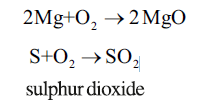Likewise, removal of oxygen is called Reduction.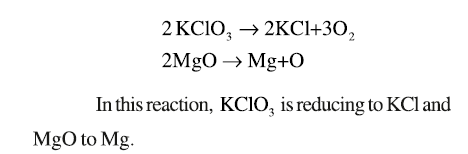Oxidation reduction on the basis of addition and removal of hydrogen: The reaction where hydrogen is removed is called Oxidation.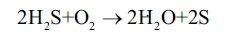Also, if the hydrogen is added in the reaction, then it is called reduction.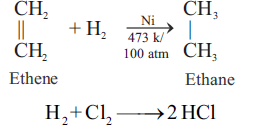Oxidation Reduction on the basis of the addition or removal of electropositive elements: If the electropositive element is removed, it is oxidation reaction, while adding the electropositive element takes place in reduction reaction.

Oxidation: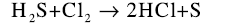Reduction: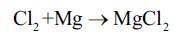Oxidation Reduction on the basis of the addition or removal of electronegative elements: If the electronegative element is added, it is oxidation reaction, while removing the electronegative element takes place in reduction reaction.

Oxidation: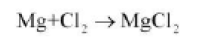Reduction: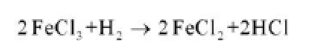Redox on the basis of Electron: The reaction in which an atom, ion or molecule donates an electron is called oxidation. One substance is reducing, while the other is oxidising. The substance that is reducing accepts the electron. Learn more with Oxidation-Reduction.

28. What do you know about the types and properties of catalysts?

Answer: Catalysts can be heterogeneous or homogeneous. Check out the type of catalysts in detail. Also, find here the properties of catalyst:

• Change in velocity. Chemical composition and amount remains constant
• Presence of catalyst in reaction mixture for a small amount is enough
• There are specific catalysts for different reactions, a single catalyst cannot work for all
• Does not initiate any reaction, only increases the velocity of reaction
• In reversible reactions, catalyst can influence the rate of both the backward and forward reaction
• They have more impact at certain temperature, with change in temperature it affects the activity of the catalyst

29. Write the steps of writing a chemical equation and its properties.

Answer: Steps to writing a chemical equation is as follows:

(i) Write the reactants, put an arrow then write the products

(ii) When there are more number of reactants or products, a + sign is placed between them. Example is given below: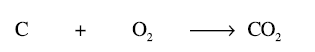(iii) In the chemical reaction, mass is neither created nor destroyed. Number of reactants and products remain constant on both sides of the equation.

(iv) Equation is balanced by the increasing and decreasing number of molecules on both sides

(v) In order to balance the equations, at first the atoms other than hydrogen or oxygen are balanced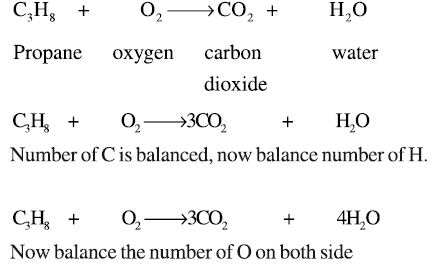(vi) After balancing the equation, represent the physical state of the reactants and products – s for solids, g for gas, l for liquid and so on.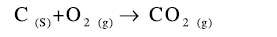(vii) When reactants and products are in aqueous form then (aq) is written.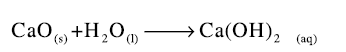(viii) When the reaction is reversible that it occurs on both sides, then the sign is used as well as the temperature and pressure required to complete the reaction is given above the arrow.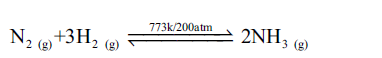(ix) For exothermic or endothermic reactions the + and – negative symbols are applied with the amount of heat on the product side. Heat is also written by Δ.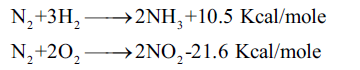(x) Catalyst used in the reaction is given above the arrow.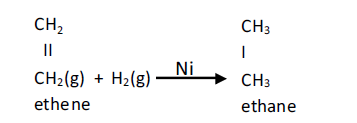Meanwhile, the properties to write the equation are also given here:

• Complete information such as the number of molecules, mass and so on of the reactants and products are obtained from the equation
• Physical state of the substance can be determined
• Required conditions for the reactions, such as the temperature, pressure, catalyst and so on are known from it
• By equation it becomes clear, if it is exothermic or endothermic
• Gives information about reversibility of the reactions

30. Write the differences between:

(a) Reversible and irreversible reactions

(b) Catalyst promoter and catalyst inhibitor

(c) Homogeneous and heterogeneous catalysis

(d) Oxidation-Reduction inhibitor

Answer: (a) Reversible and irreversible reactions

A reversible reaction moves in both directions, while an irreversible reaction occurs only in forward direction.

(b) Catalyst promoter and catalyst inhibitor

A catalyst promoter accelerates the rate of chemical reaction, while the catalyst inhibitor reduces the speed of reaction.

(c) Homogeneous and heterogeneous catalysis

In a homogeneous catalyst, the physical state of the catalyst, reactants and products is the same. If in a chemical reaction, the physical state of the catalyst and the reactants are different, then the catalyst is a heterogeneous catalyst.

(d) Oxidation-Reduction

Adding oxygen to a substance is oxidation, while removing oxygen from a substance is reduction.

### RBSE Class 10 Science Chapter 6 Additional Questions-Important Questions and Solutions

31. How to chemically remove the black coating of copper oxide?

Answer: To remove the black coating of copper oxide chemically, pass hydrogen gas over heated copper oxide. The black coating turns brown as oxygen is removed by hydrogen.

32. When quick lime is added to water a hissing sound is produced. What is the chemical reaction and mention the type of reaction taking place.

Answer: CaO + H2O → Ca(OH)2+ Δ

It is an exothermic and combination reaction.

33. Explain why most of the metal articles become dull when kept exposed to air?

Answer:  The surface of metal articles when it reacts with the gases present in the air gets coated with the layer of compound it forms thus making the metal look dull. Thus, the metals lose lustre. For example, aluminium metal reacts readily with oxygen to create aluminium oxide and its surface becomes dull.

34. Write the differences between the exothermic and endothermic reactions.

Answer: In Exothermic reaction, heat is given out during the reaction, while in Endothermic reaction heat is taken in to break the bonds and to form new compounds.

35. Photosynthesis is considered as an endothermic reaction. Why?

Answer: In Photosynthesis reaction, energy is required to produce glucose from carbon dioxide and water. Energy in the form of sunlight is needed to break the bonds of hydrogen and oxygen. For this reason, it is known as endothermic reaction.

36. Define electrolytic decomposition. Mention two uses of electrolytic decomposition reaction.

Answer: When electricity is passed through a molten compound that is ionic in nature, then then the ions of the compound separates into its components, thus decomposing the compound. It is used to separate hydrogen and oxygen gas from water. It is also used to separate gases or metals from the compound.

37. How is a chemical equation balanced?

Answer: The number of atoms on the left hand side of the reaction that is for the reactants is equal to the number of atoms on the right hand side of the equation (i.e., products).

38. When a magnesium ribbon burns in air with a dazzling flame and forms a white ash, is magnesium oxidised or reduced? Why?

Answer: The redox reaction here is.

2Mg + O2 → 2MgO

In this case, magnesium is oxidised when the oxygen is combined with it.

39. Mention some examples of exothermic reaction.

Answer: Respiration and water added to lime are examples given for exothermic reactions

40. GIve the name of a reducing agent that may be used to obtain manganese from manganese dioxide.

Answer: Coke or carbon is used as a reducing agent to obtain manganese from manganese dioxide.

After reading through Rajasthan Board 10 Class Science Book, students must attempt the Rajasthan Board Class 10 previous years question papers to understand the RBSE Science paper pattern.